Puzzle Demo

Demos > Puzzle Demo

This is an example showing how to implement a simple puzzle game with Sortables.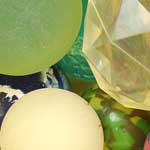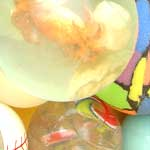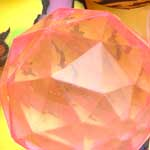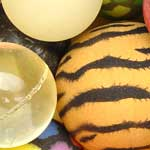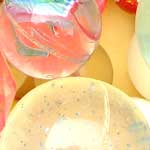Source code

(function() {
var p = \$('puzzle'), info = \$('puzzleinfo'), moves = 0;

Sortable.create('puzzle', {
tag: 'img', overlap: 'horizontal', constraint: false,
onUpdate: function() {
info.update('You\'ve made ' + (++moves) + ' move' + (moves > 1 ? 's' : ''));
if (Sortable.sequence('puzzle').join('') == '123456789')
info.update('You\'ve solved the puzzle in ' + moves + ' moves!').morph('congrats');
}
});
})();